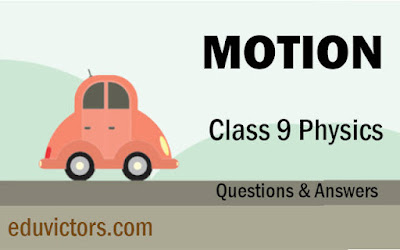## Pages

Showing posts with label class9-physics. Show all posts
Showing posts with label class9-physics. Show all posts

# CBSE Class 9 Physics - Sound - Very Short Answer-Based Questions (VSQA)

Q1. Define vibration?

Answer: Rapid to and fro motion of an object.

Q2. How is sound produced?

Q3. Define wave.

Answer: A wave is a disturbance produced in a medium as the particles of the medium vibrate.# Class 9 - Physics - What is the difference between Gravitation Constant (G) and Gravitational acceleration (g)

The gravitational constant (G) is a fundamental physical constant that appears in Newton's law of universal gravitation. It determines the strength of the gravitational force between two objects, and it is always the same, regardless of the distance between the objects or their masses. The value of G is approximately 6.674 x 10⁻¹¹ N(m/kg)².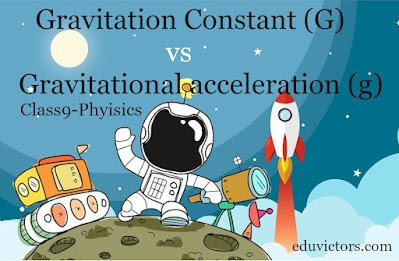# CBSE Class 9 - Physics - Work (Questions and Answers)

Q1: 2 Newton-metre is how many joules?

Answer: ∵ 1 Nm = 1J ∴ 2Nm = 2J

Q2: In the CGS system, what is the unit for Work?# Class IX Science Term 1 MCQs (2021-22)

Q1. Rusting of an article made up of iron is called

(a) corrosion and it is a physical as well as chemical change

(b) dissolution and it is a physical change

(c) corrosion and it is a chemical change

(d) dissolution and it is a chemical change# Gravitation 1 - Mark Questions (Solved) CBSE Class 9 - Physics

Q1: Who formulated the universal law of Gravitation?

Q2: What is free fall?

Answer: When an object falls from any height under the influence of gravitational force only, it is known as a free fall.

Q3: What is the value of gravitational constant G on the earth? Does the value G change on the moon?

Answer: The value of the gravitational constant on the earth is 6.67×10⁻¹¹ Nm²/kg². The value of G does not change (universal constant), it remains the same on the moon.

Q4: What will happen to the gravitation force between two bodies, if the mass of one body is doubled?

Answer: Gravitational force will double also.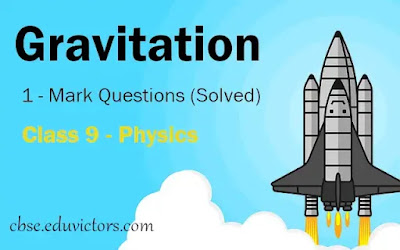## Chapter Motion: Class 9 - Physics - Short Questions and Answers - Part-1

Q1:  An object has moved through a distance. Can it have zero displacements? If yes, support your answer with an example.

Answer: Yes, even if an object has moved through a distance, it can have zero displacements. For example, an object after moving through a certain distance (say d), comes back to its starting position. In that case, the distance covered is 2d while displacement is zero.

Q2: Is displacement a scalar quantity?

Answer: No, displacement is a vector quantity because it has magnitude as well as direction.

Q3:  State whether the distance is a scalar or a vector quantity.

Answer: Distance is a scalar quantity; it has magnitude but no specific direction.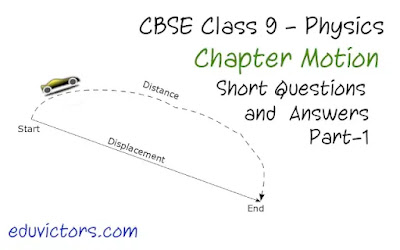# Physics: Important Discoveries

No. Discovery Scientist Year
1. Proton Rutherford 1919
3. Atom John Dalton 1808
4. Atomic Structure Neil Bohr & Rutherford 1913
7. Raman Effect CV. Raman 1928
8. X-Rays Roentgen 1895
9. Photoelectric effect Albert Einstein 1905
10. Periodic table Mendeleev 1888
11. Nuclear Reactor Anrico Fermi 1942
12. Wireless Telegram Marconi 1901
13. Dynamite Alfred Nobel 1867## Monday 31 December 2018

### CBSE Class 9 - Physics - Work, Power and Energy - Worksheet (#eduvictors)(#cbsenotes)

Class 9 - Physics

## Work, Power and Energy

Worksheet

Fill in the blanks with suitable words.

1. Energy is the __________ to do work. Its S.I. unit is ________.

2. Work is force multiplied by ___________ in the direction of force.

3. If the angle between force and the displacement caused is acute then work is said to be ________.

4. If the angle between force and the displacement caused is obtuse, the work is said to be _______.

5. One joule of work is said to be done when a force of ______ displaces a body through a distance of ________ in its own direction.## CBSE Class 9 - Physics -Gravitation and Flotation (Solved Numerical Problems)

Questions:

1) Find the total thrust acting on the bottom surface of a tank 4m long, 2m broad and 2m deep when fully filled with water. The density of water= 10³ kg/m³.

2) The gravitational force between two objects is 100N. How should the distance between the objects be changed so that force between them becomes 50N?

3) If the distance between two masses be increased by a factor of 6, by what factor would the mass of one of them have to be altered to maintain the same gravitational force? Would this be an increase or decrease in the mass?

4) The value of ‘g’ on earth’s surface is 9.8 m/s². Suppose the earth suddenly shrinks to one-third of its present size without losing any mass. What is the value of ‘g’ on the surface of shrunk earth?

5) Two bodies of mass 1kg and 100kg respectively are dropped simultaneously from same height 4.9m in a vacuum. Calculate and compare their final velocities just before hitting the ground and the time interval in which they will hit the ground.

6) The ratio of gravitational force on Neptune to the gravitational force on earth is 9:8. Ramesh weighs 792N on earth. Calculate his weight on Neptune.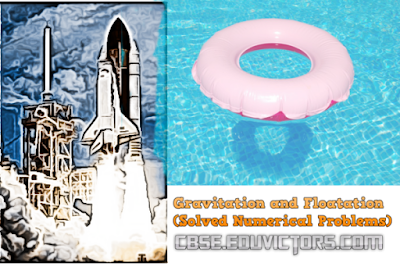# Motion

#### NCERT Chapter Questions and Answers and other Q & A

Q1: An object has moved through a distance. Can it have zero displacements? If yes, support your answer with an example.

Answer: Yes an object can have zero displacements even though it has moved through a distance. It happens when the object moves back to its original position i.e. final position coincides with the starting position.

Example: Suppose an object travels from O to C and then comes back to its original position O.
Total distance traveled = actual path covered = OC + CO = 25 + 25 = 50m
Total displacement = shortest distance between final position and initial position = 0m

Q2: What do you mean by a body in rest?
Answer: A body is said to be at rest if it does not change its position with respect to a fixed point in its surroundings.

Q3: Are motion and rest absolute or relative? Explain with an example.
Answer: No these terms rest and motion are relative. For example, a person inside a car, carrying a ball in his hand will see the ball is at rest. While for another person, outside the car will see the ball is also moving.

Q4: What is meant by scalars and vectors?
• Scalar Quantities: Quantities that require magnitudes only to specify them are called scalar quantities or scalars. Mass, length, time, temperature, angle, area, speed, distance, volume and density are examples of scalar quantities.
• Vector Quantities: Quantities that require both magnitudes and direction to specify them are called vector quantities or vectors. Displacement, velocity, force, momentum, weight etc. are examples of vectors.
Q5: A farmer moves along the boundary of a square field of side 10 m in 40 s. What will be the magnitude of displacement of the farmer at the end of 2 minutes 20 seconds?

As shown in the figure, let us assume, that the farmer starts from A.
Given, that the length of each side = 10m
Distance covered in 1 lap = Perimeter of ABCD = 4 x 10 = 40m
Time is taken by farmer to cover 1 lap = the 40s
Speed of farmer = Distance ÷ Time Taken for one lap = 40/40s = 1m/s

Distance covered by farmer in 2min 20 secs = Speed x Time = 1 x 140s = 140m

Number of laps covered = 140 ÷ 40 = 3.5 laps.
⇒ After the 140s, the farmer will be at position C (i.e. 3 and ½ laps).

Displacement = AC = (AB2 + BC2)½                 (applying Pythagoras theorem)
= (100+100)½ = 10√2 = 10 x 1.414 = 14.14m      .... (answer)

Question: Can you find out displacement, if the farmer starts from the mid-point of AB?

Note: Displacement is a vector quantity that measures the shortest distance (straight line) between the starting point and ending point, not taking the actual path travelled into account.